American Journal of Modern Energy
Volume 2, Issue 3, June 2016, Pages: 10-16

Analysis of Wind Speed Distribution: Comparative Study of Weibull to Rayleigh Probability Density Function; A Case of Two Sites in Ethiopia

Girma Dejene Nage

Department of Physics, College of Natural and Computational Sciences, Mizan-Tepi University, Tepi, Ethiopia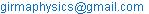Girma Dejene Nage. Analysis of Wind Speed Distribution: Comparative Study of Weibull to Rayleigh Probability Density Function; A Case of Two Sites in Ethiopia. American Journal of Modern Energy. Vol. 2, No. 3, 2016, pp. 10-16. doi: 10.11648/j.ajme.20160203.11

Received: June 30, 2016; Accepted: July 28, 2016; Published: August 26, 2016

Abstract: This study is primarily aimed to estimate the wind speed distribution of the location, Dire Dawa and Hawasa. Wind speed is the most important parameter in the analysis of wind energy resource, design and study of wind energy conversion systems. In this study, statistical methods were used to analyze wind speed data of Dire Dawa and Hawasa. Based on these data, the shape, k and scale, c parameters of the two locations were determined. The monthly mean values of k range from 1.86 to 8.19, with yearly mean value of 4.46, while c is in the range of 1.60 to 3.65 m/s with yearly mean value of 2.59 m/s for Dire Dawa and monthly mean values of k range from 2.00 to 2.79, with yearly mean value of 2.38, while c is in the range of 1.50 to 2.19 m/s with yearly mean value of 1.76 m/s for Hawasa. Two probability density functions are fitted to the measured probability distributions on a monthly basis. From statistical analysis of distributions, the Weibull distribution is better in fitting the measured probability density distributions than the Rayleigh distribution for the whole year. The cumulative probability distribution indicates, the probability for which the wind blows with a monthly mean wind speed v is equal or lower than 5 m/s is almost one.

Keywords: Weibull Distribution, Rayleigh Distribution, Wind Speed Distribution, Weibull Parameters

Contents

1. Introduction

Wind energy as a clean, environmentally friendly and cost effective renewable energy resource, is taken into consideration by many developed and developing countries as a promising means to provide electrical energy. Ethiopia needs substantial amount of energy to develop its industry and to increase the productivity. Ethiopia has exploitable potential of energy resources, more than 10,000 MW from wind . Currently, four wind farms are under construction and one wind farm with capacity of 52 MW power already completed in December 2012. Lack of organized data on the energy potential of the country covering the entire regions has been one of the reasons for limited application of wind energy in Ethiopia [1,2]. Therefore, effective utilization of wind energy entails a detailed knowledge of the wind characteristics at the particular location. It is not an easy task to choose a site where wind energy conversion systems may be installed because many factors have to be taken into account. The most important factors are the wind speed, the energy of the wind, the generator type and a feasibility study .

The energy available in the wind varies as the cube of the wind speed, so an understanding of the characteristics of the wind resource is critical to all aspects of wind energy exploitation, from the identification of suitable sites and predictions of the economic viability of wind farm projects through to the design of wind turbines themselves, and understanding their effect on electricity distribution networks and consumers. From the point of view of wind energy, the most striking characteristic of the wind resource is its variability. The wind is highly variable, both geographically and temporally. Furthermore this variability persists over a very wide range of scales, both in space and time. Consequently, due to limited and lack of organized data, probabilistic distribution of wind speed is one of critical piece of information needed in the assessment of wind energy potential. This study addresses analysis of wind speed probability distributions: comparative study of Weibull to Rayleigh distribution function; a case of two sites in Ethiopia.

2. Wind Velocity Distribution

Being a stochastic phenomena, speed and direction of wind varies widely with time. Apart from the seasonal variations, the differences can be considerable even within a short span of time . These variations can significantly affect the energy yield from the turbine at a given site. In literature there are statistical distributions most suitable for defining the characteristics of wind regime.

2.1. Weibull Distribution

The conventional (two-parameter) Weibull probability density function has widely been used for describing wind regimes written as [4,13,16,22]: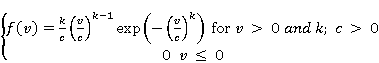(1)

where v is the wind speed; k is the shape parameter (dimensionless) and c is the scale parameter having the same dimension as wind speed. Note that the Weibull distribution is related to a number of other probability distributions; in particular, it becomes the exponential distribution (if shape parameter k = 1).

The Weibull cumulative distribution function is given by [4,5,16,22](2)

There are several methods for determining k and c from the site wind data. Some of the common methods are the graphical method, moment method, maximum likelihood method, energy pattern factor method and the standard deviation method [4-6,13,15,16]

The standard deviation approach: the relationship between the mean (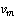) and standard deviation (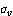) of the wind velocities and k are correlated as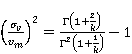(3)

From this equation k can be determined by solving the equation numerically. Then, once k is determined, c is given by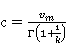(4)

In a simpler approach, an acceptable approximation for k can be made as [8,9,13]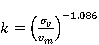(5)

Similarly, c can be approximated as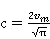(6)

More accurately, c can be found using the expression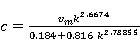(7)

The moment method: In this method, the nth moment Mn of Weibull distribution is given by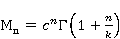(8)

If M1 and M2 are the first and second moments, with Eq. (8), c can be solved as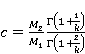(9)

Likewise,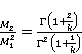(10)

In this method, M1 and M2 are calculated from the given wind data. Further, k and c are estimated by solving Eq. (9) and Eq. (10).

The maximum likelihood method: In the maximum likelihood method, the shape and scale factors are given as [6,7,11,14]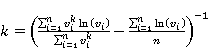(11)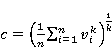(12)

where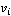is the wind speed in time step i and n is the number of data points.

Least-squares regression method: In this method, the cumulative distribution function is transformed into a linear form, adopting logarithmic scales [9,12,13]. Then, the expression for the cumulative distribution of wind velocity can be rewritten as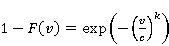(13)

Taking the logarithm twice, we get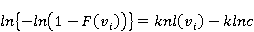(14)

Plotting the above relationship with ln (Vi) along the x- axis and ln{-ln [1-F (Vi)]} along the y- axis, nearly a straight line is obtained. From Eq. (14), k gives the slope of this line and –klnc represents the intercept.

The cumulative distribution function F (v) can be estimated easily, using an estimator, which is the median rank, according to Benard’s approximation :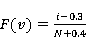(15)

where i is the number of the wind speed measurements and N is the total number of observations.

Energy pattern factor method: Energy pattern factor (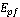) is the ratio between the total power available in the wind and the power corresponding to the cube of the mean wind speed . Thus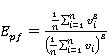(16)

Once the energy pattern factor for a regime is found from the wind data, an approximate solution for k is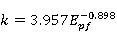(17)

As the scale and shape parameters have been calculated, two meaningful wind speeds for wind energy estimation, the most probable wind speed and the wind speed carrying maximum energy, can be easily obtained. The most probable wind speed denotes the most frequent wind speed for a given wind probability distribution and is expressed by [4,11]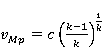(18)

The wind speed carrying maximum energy represents the wind speed that carries the maximum amount of wind energy and is expressed as follows [4, 11]: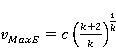(19)

2.2. Rayleigh Distribution

This is the simplest wind speed probability distribution to represent the wind resource since it requires only a knowledge of the mean wind speed,. It is a special case of Weibull distribution (when k=2). The probability density function and the cumulative distribution function are given by [4,13]: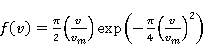(20)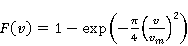(21)

3. Suitability Judgment Criteria

Celik  conducted a literature survey and found that the judgment of the suitability of a possible distribution function was made only based on wind speed distribution. The criteria include the mean wind speed, standard deviation or the coefficient of determination (COD)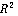of the wind speed distribution and direct comparison of the model parameters.

The coefficient of determination (COD) is used to evaluate the performance of the MEP-based distributions, the Weibull and Rayleigh distributions. This coefficient expressed as a percentage indicates how much of the total variation in the dependent variable can be accounted for by the theoretical or empirical distribution. A higher COD represents a better fit using the theoretical or empirical function. A complete fit has the COD; value of 100 %. The COD is defined as [16,17-19].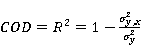(22)

where R is the correlation coefficient [19,21] and σy is the standard deviation of the measured data y from its own mean value ym, and is conventionally defined as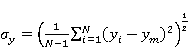(23)

and similarly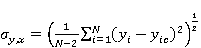(24)

where yi are the actual values of y as measured, and yic are the values computed from the correlation equation for the same independent value of x. In the present context, y represents the probability density, f (V), while the wind speed acts as the independent parameter x.

Moreover, chi-square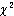and root mean square error analysis (RMSE) were used as the primary criterion to select the best distribution to account for the variation in the wind speed curves. Chi-square is the mean square of the deviations between the experimental and calculated values for the distributions and was used to determine the goodness of the fit. The lower are the values of chi-square, the better is the goodness of the fit. The RMSE gives the deviation between the predicted and experimental values, and it is required to approach zero [23,24]: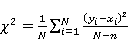(25)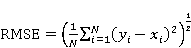(26)

where yi is the ith experimental data, ym is the mean value of the experimental data, xi is the ith predicted data with the Weibull or Rayleigh distribution, N is the number of observations and n is the number of constants.

4. Results and Discussion

In this study, the wind speed data which were recorded in three hour time interval for both Dire Dawa (9.600°N; 41.867°E; 1276 m) and Hawasa (7.050°N; 38.467°E; 1708 m) that measured from 2003 to 2005, have been statistically analyzed. The wind speed data of the two sites were obtained from National Meteorological Agency of Ethiopia. Based on these data, the wind speeds were analyzed and processed using spreadsheet and MATLAB computer software. The main results obtained from this study can be summarized as follows:

4.1. Wind Characteristics and Weibull Parameters

The monthly mean wind speed valuesand Weibull parameters k and c are presented in Table 1 and Table 2 for both sites, 2003–2005. The monthly mean wind speed values are between 1 and 4 m/s for Dire Dawa and range from 1 to 2 m/s for Hawasa. While June of Dire Dawa has the highest mean wind speed value with 3.46 m/s and November exhibits the minimum monthly mean wind speed value of 1.42 m/s. Hawasa has monthly mean wind speed value of minimum 1.34 m/s and highest 1.94 m/s for January and June respectively.

Similarly, values of the two Weibull parameters, the scale parameter c (m/s) and shape parameter k (dimensionless), calculated from the long term wind data for the site studied. It is shown that the parameter k has a much smaller, temporal variation for Hawasa than Dire Dawa site. The monthly average values of k and c range between 1.86 - 8.19 and 1.60-3.65 m/s with yearly an average value of 4.64 and 2.59 m/s for Dire Dawa, respectively and range between 2.00 – 2.79 and 1.50 -2.19 m/s with yearly an average value of 2.38 and 1.76 m/s for Hawasa, respectively.

Table 1. Monthly wind characteristics: mean wind speed, the most probable wind speed, the wind speed carrying maximum energy, k and c for Dire Dawa site, 2003-2005.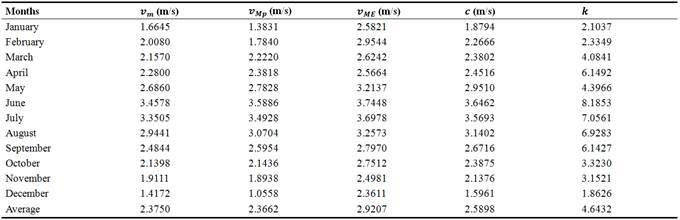Table 2. Monthly wind characteristics: mean wind speed, the most probable wind speed, the wind speed carrying maximum energy, k and c for Hawasa site, 2003-2005.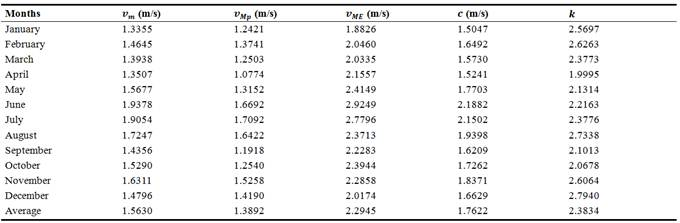It is clear from Table 1, 2 and illustration in Fig. 1 and 2, Dire Dawa has a significant seasonal and diurnal wind speed variation than Hawasa. The diurnal variation of the wind speed at Hawasa in January, May, July and October as it is seen from Fig. 2, wind speed has its minimum during the morning hours and maximum during the afternoon hours.Figure 1. Diurnal variation of wind speed for some months, Dire Dawa: January, May, July and October.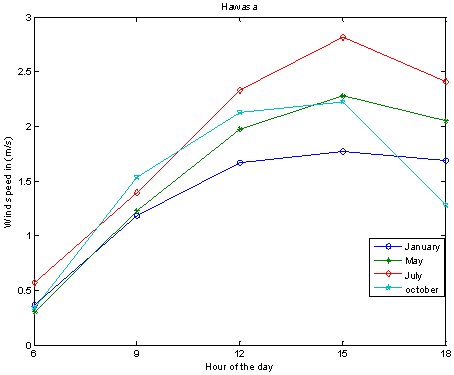Figure 2. Diurnal variation of wind speed for some months, Hawasa: January, May, July and October.

4.2. Comparison of Weibull and Rayleigh Distributions

For the purpose of comparison and fitted to measured data, one month from each four seasons was considered, since the characteristics of wind in months of each season shows similar trend in the location under study. In general, the values of the scale parameters are low during the winter and autumn and high during the summer for Dire Dawa and low during the spring and high during the summer for Hawasa.

The Weibull and Rayleigh approximations of the actual probability density distribution of wind speeds for some months in each season in the year are shown in Fig. 3 and 4, while a comparison of the two approximations with the actual probability distribution is given in Table 3. As it can be seen in Table 3, the highest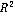value was obtained by using the Weibull distribution. Likewise, the results have shown that the RMSE and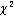values of the Weibull distribution are lower than the values obtained by the Rayleigh distribution. As result, the Weibull approximation is found to be the most accurate distribution according to the highest value ofand the lowest values of RMSE andfor both sites.Figure 3. Weibull and Rayleigh approximations of the actual probability distribution of wind speeds, Dire Dawa.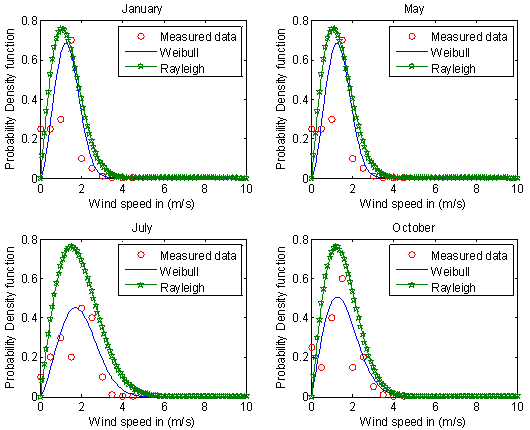Figure 4. Weibull and Rayleigh approximations of the actual probability distribution of wind speeds, Hawasa.

Table 3. Comparison of Weibull and Rayleigh probability density for some months via statistical analysis parameters: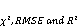.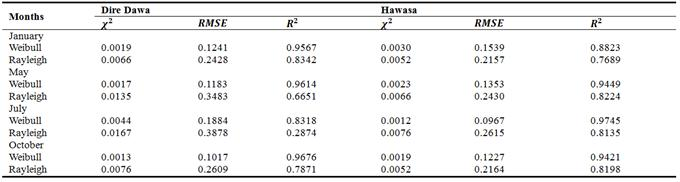Furthermore, cumulative probability distributions of wind speeds are shown in Fig. 5 and 6, which indicates the fraction of time (or probability) that the wind speed is equal or lower than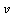. The cumulative distribution function can also be used for estimating the time for which wind is within a certain speed interval. It can be seen from Fig. 5 and 6, the probability for which the wind blows with a mean speed v is equal or lower than 5 m/s is almost one.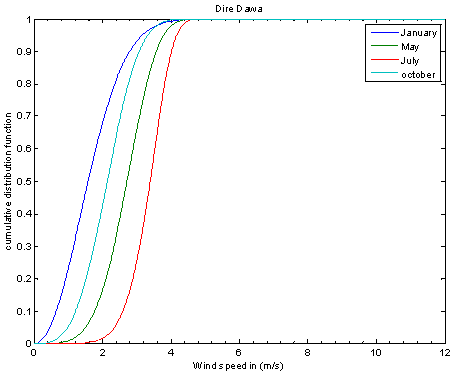Figure 5. Monthly cumulative density functions derived from diurnal wind speed data, Dire Dawa.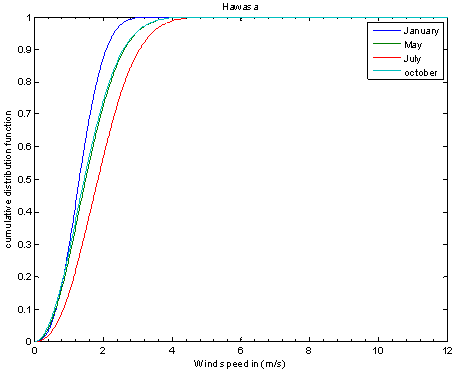Figure 6. Monthly cumulative density functions derived from diurnal wind speed data, Hawasa.

5. Conclusions

In this study, wind speed data of Dire Dawa and Hawasa have been statistically analyzed. The probability density distributions have been derived for wind speed data and the distributional parameters were identified. Two probability density functions have been fitted to the measured probability distributions on a monthly basis. The most important outcomes of the study can be summarized as follows:

Dire Dawa has a considerable, seasonal and diurnal wind speed variation than Hawasa.

Wind characteristics for both sites were investigated, on monthly basis, mean wind speed, the most probable wind speed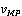, the wind speed carrying maximum energy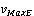and Weibull parameters.

The values of the shape parameter, k and scale parameter, c for Dire Dawa and Hawasa were examined and the yearly average found to be 4.64 and 2.38 for k and 2.59 m/s and 1.76 m/s for c, respectively.

The Weibull distribution is better in fitting the measured probability density distributions than the Rayleigh distribution for the whole year. This is obvious from the statistical analysis of distributions.

It is evident from cumulative probability distribution, the probability for which the wind blows with a monthly mean wind speed v is equal or lower than 5 m/s is almost one.

This study is primarily aimed to estimate the wind speed distribution of the location, Dire Dawa and Hawasa and identify the better distribution model. However, to study wind energy potential and power density analysis, it is better to have a comprehensive treatment of Weibull and the Maximum entropy principle distribution.

References

1. Ethiopian Electric Power Corporation (EEPCo), 2011. Strategic management and programming; Facts in brief.
2. Ethiopian Electric Power Corporation (EEPCo), 2011. Strategic plan 2011–2025. Addis Ababa.
3. Kose R., 2003. An evaluation of wind energy potential as a power generation source in Kutahya, Turkey. Energy Conversion and Management, 45:1631–1641.
4. Mathew S., 2006. Wind Energy: Fundamentals, Resource Analysis and Economics. Springer-Verlag, Berlin Heidelberg.
5. Chang TP., 2011. Estimation of wind energy potential using different probability density functions. Appl Energy; 88:1848–56.
6. Chang TP., 2011. Performance comparison of six numerical methods in estimating Weibull parameters for wind energy application. Appl Energy; 88:272–82.
7. Seguro JV, Lambert TW., 2000. Modern estimation of the parameters of the Weibull wind speed distribution for wind energy analysis. J Wind Eng Ind. Aerodynamics; 85:75–84.
8. Boweden GJ, Barker PR, Shestopal VO, Twidell JW., 1983. The Weibull distribution function and wind statistics. Wind Engineering 7:85-98.
9. Justus CG, Hargraves WR, Mikhail A, Graber D., 1978. Methods of estimating wind speed frequency distribution. J Applied Meteorology 17:350-353.
10. Ananstasios B, Dimitrios C, Thodoris DK, 2002. A nomogram method for estimating the energy produced by wind turbine generators. Solar Energy 72(3): 251-259.
11. Chang TJ, Wu YT, Hsu HY, Chu CR, Liao CM, 2003. Assessment of wind characteristics and wind turbine characteristics in Taiwan. Renewable Energy 28:851-871.
12. Garcia A, Torres JL, Prieto E DE, Francisco A.,1998. Fitting wind speed distributions: a case study. Solar Energy 62(2): 139-144.
13. Manwell J. F, Mcgowan J. G and Rogers A.L, 2009. Wind energy explained: Theory, design and application. 2nded., John Wiley & Sons Ltd.
14. Stevens MJM, Smulders PT., 1979. The estimation of parameters of the Weibull wind speed distribution for wind energy utilization purposes. Wind Engineering 3(2): 132-145.
15. Twidell J. W and Weir A.D, 2006. Renewable Energy Resources. 2nded., Taylor & Francis.
16. Li X, 2011. Green Energy: Basic Concepts and Fundamentals, Springer-Verlag London Limited.
17. Li M., Li X., 2005. MEP-type distribution function: a better alternative to Weibull function for wind speed distributions. Renewable Energy 30: 1221–1240.
18. Ramirez P, Carta JA., 2006. The use of wind probability distributions derived from the maximum entropy principle in the analysis of wind energy. A case study. Energy Convers & Manage; 47: 2564–77.
19. Akpinar S., Kavak Akpinar E., 2007. Wind energy analysis based on maximum entropy principle (MEP)-type distribution function; Energy Convers & Manage 48:1140–1149
20. Celik AN., 2003. Assessing the suitability of wind speed probability distribution functions based on wind power density. Renewable Energy; 28: 1563–74.
21. Holman JP, Gajda Jr WJ, 1984. Experimental methods for engineers. 4th ed.; McGraw-Hill.
22. Carta JA, Ramirez P., 2007. Use of finite mixture distribution models in the analysis of wind energy in the Canarian Archipelago. Energy Convers Manage;48:281–91.
23. Akpinar S., Kavak Akpinar E., 2005.A statistical analysis of wind speed data used in installation of wind energy conversion systems. Energy Convers & Manage 46: 515–532.
24. Holman JP., 1971. Experimental Methods for Engineers. New York: McGraw-Hill Book Company; p. 37–52.
25. Benard, A., Bos-Levenbach, E C., 1953. Het uitzetten van waarnemingen op waarschijnlijkdeidspapier (the plotting of observations on probability paper); Statististica Neerlandica, 7, 163–173.

 Contents 1. 2. 2.1. 2.2. 3. 4. 4.1. 4.2. 5.
Article ToolsAbstractPDF(316K)